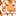# QlikView App Dev

Discussion Board for collaboration related to QlikView App Development.

Announcements
Action-Packed Learning Awaits! QlikWorld 2023. April 17 - 20 in Las Vegas: REGISTER NOW
cancel
Showing results for
Did you mean:Creator

## Nested if in load script error

[ ID Key],

CPKey,

Sum(IF(sum(if(CYQ='Q'&ceil(Month(Today())/3)&'_'& year(Today()),Comm)) <=0,

sum(if(CPYQ='Q'&ceil(Month(Today())/3)&'_'& year(Today()),CommPlan)),

sum(if(CYQ='Q'&ceil(Month(Today())/3)&'_'& year(Today()),Comm)))) as SF

Resident SF

Group by [ID Key],

CPKey;

Drop Table SF;

I a using the above script and I am getting error saying cannot do nested aggregation is not allowed ? Cant I do it or I am doing something wrong here ?

1 Solution

Accepted SolutionsMVP

May be this

CPKey,

If(Sum(if(CYQ='Q'&ceil(Month(Today())/3)&'_'& year(Today()),Comm)) <= 0,

Sum(if(CPYQ='Q'&ceil(Month(Today())/3)&'_'& year(Today()), CommPlan)),

Sum(if(CYQ='Q'&ceil(Month(Today())/3)&'_'& year(Today()),Comm))) as SF

Resident SF

Group by [ID Key], CPKey;

DROP Table SF;

4 RepliesMVP

May be this

CPKey,

If(Sum(if(CYQ='Q'&ceil(Month(Today())/3)&'_'& year(Today()),Comm)) <= 0,

Sum(if(CPYQ='Q'&ceil(Month(Today())/3)&'_'& year(Today()), CommPlan)),

Sum(if(CYQ='Q'&ceil(Month(Today())/3)&'_'& year(Today()),Comm))) as SF

Resident SF

Group by [ID Key], CPKey;

DROP Table SF;Creator
Author

How Silly..I just missed the if !!

Thank you very much.You are a rockstar !!MVP

You didn't miss an if... but you had an extra Sum()Creator
Author

Yup !!Community Browser